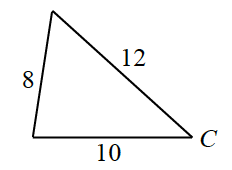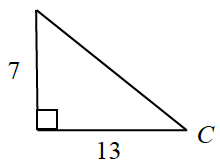### Home > CCA2 > Chapter Ch12 > Lesson 12.2.1 > Problem12-105

12-105.

Find $m∠C$ in each triangle below.

1.Use the Law of Cosines to solve this problem.

$8^2 = 12^2 + 10^2 - 2(12)(10)$cos$(C)$

$41.41°$

1.This is a right triangle, and you know the lengths of both legs. Which trig function uses this information?